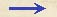Discorsi Propositions 2/31-th-20-lemma Discorsi Proposition2/31-th-20-lemmaLEMMA. LEMMASi duo circuli se se intus contingant, quorum interiorem quaelibet linea recta contingat, exteriorem vero secet, tres lineae a contactu circulorum ad tria puncta rectae lineae tangentis, nempe ad contactum interioris {30} circuli et ad sectiones exterioris, protractae, angulos in contactu circulorum aequales continebunt. If two circles one lying within the other are in contact, and if any straight line be drawn tangent to the inner circle, cutting the outer circle, and if three lines be drawn from the point at which the circles are in contact to three points on the tangential straight line, namely, the point of tangency on the inner circle and the two points where the straight line extended cuts the outer circle, then these three lines will contain equal angles at the point of contact. Tangant se intus in puncto A duo circuli, quorum centra, B minoris, C maioris; {253} interiorem vero circulum contingat recta quaelibet linea FG in puncto H, maiorem autem secet in punctis F, G; et connectantur tres lineae AF, AH, AG: dico, angulos ab illis contentos FAH, GAH esse aequales. Extendatur AH usque ad circumferentiam in I, et ex centris producantur BH, CI, et per eadem centra ducta sit BC, quae extensa cadet in contactum A et in circumferentias circulorum in O et N: et quia anguli ICN, HBO aequales sunt, cum quilibet ipsorum duplus sit anguli IAN, erunt lineae BH, CI parallelae. Cumque BH, ex centro ad contactum, sit perpendicularis ad FG, erit quoque ad eandem perpendicularis CI et arcus FI arcui IG aequalis, {10} et, quod consequens est, angulus FAI angulo IAG. Quod erat ostendendum. Let the two circles touch each other at the point A, the center of the smaller being at B, the center of the larger at C. Draw {253} the straight line FG touching the inner circle at H, and cutting the outer at the points F and G; also draw the three lines AF, AH, and AG. Then, I say, the angles contained by these lines, FAH and GAH, are equal. Prolong AH to the circumference at I; from the centers of the circles, draw BH and CI; join the centers B and C and extend the line until it reaches the point of contact at A and cuts the circles at the points O and N. But now the lines BH and CI are parallel, because the angles ICN and HBO are equal, each being twice the angle IAN. And since BH, drawn from the center to the point of contact is perpendicular to FG, it follows that CI will also be perpendicular to FG and that the arc FI is equal to the arc IG; consequently the angle FAI is equal to the angle IAG. Q. E. D.Discorsi Propositions 2/31-th-20-lemma Discorsi Proposition2/31-th-20-lemma